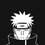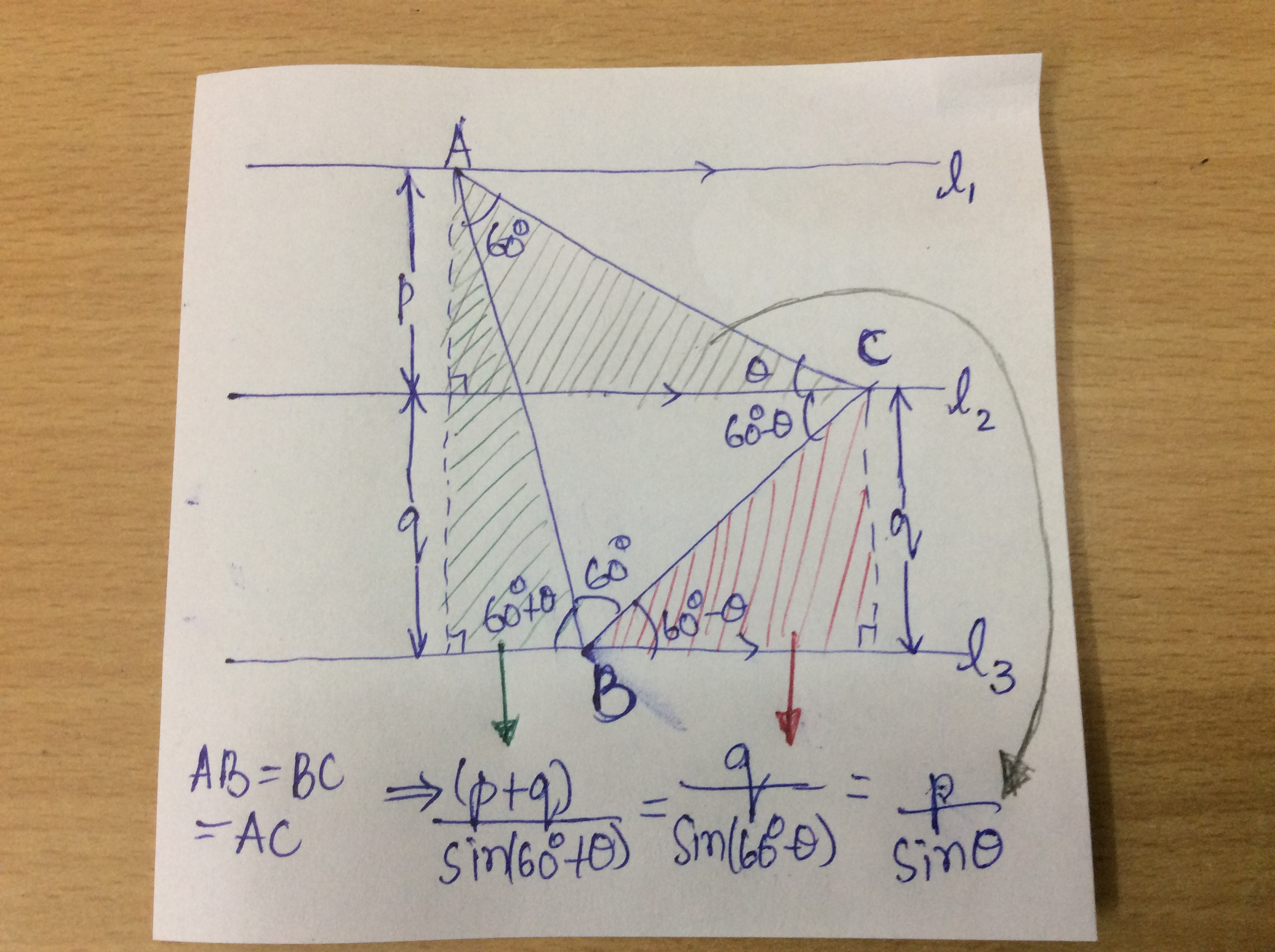# Questionnaire

Happy Problem solving !!

$1)$ Prove that in a right triangle, the bisector of right angle halves the angle between the median and the altitude drawn from the same vertex.

$2)$ Prove that if in a triangle, the ratio of tangents of two angles is equal to the ratio of squares of the sines of these angles, then the triangle is either an isosceles or a right one.

$3)$ Straight lines $l_{1},l_{2},l_{3}$ are parallel, $l_{2}$ lying between $l_{1}$ and $l_{3}$ at a distance of $p$ and $q$ from them. Find the size of a regular triangle whose vertices lie on the given lines (one on each line).

$4)$ The diagonal of a rectangle divides its angle in the ratio $m:n$. Find the ratio of the perimeter of the rectangle to its diagonal.

$5)$ The acute angle of a parallelogram is equal to $\alpha$ and the sides are $a$ and $b$. Find the tangent of the acute angles formed by the larger diagonal and the sides of the parallelogram.Note by Akshat Sharda
5 years, 8 months ago

This discussion board is a place to discuss our Daily Challenges and the math and science related to those challenges. Explanations are more than just a solution — they should explain the steps and thinking strategies that you used to obtain the solution. Comments should further the discussion of math and science.

When posting on Brilliant:

• Use the emojis to react to an explanation, whether you're congratulating a job well done , or just really confused .
• Ask specific questions about the challenge or the steps in somebody's explanation. Well-posed questions can add a lot to the discussion, but posting "I don't understand!" doesn't help anyone.
• Try to contribute something new to the discussion, whether it is an extension, generalization or other idea related to the challenge.

MarkdownAppears as
*italics* or _italics_ italics
**bold** or __bold__ bold
- bulleted- list
• bulleted
• list
1. numbered2. list
1. numbered
2. list
Note: you must add a full line of space before and after lists for them to show up correctly
paragraph 1paragraph 2

paragraph 1

paragraph 2

[example link](https://brilliant.org)example link
> This is a quote
This is a quote
    # I indented these lines
# 4 spaces, and now they show
# up as a code block.

print "hello world"
# I indented these lines
# 4 spaces, and now they show
# up as a code block.

print "hello world"
MathAppears as
Remember to wrap math in $$ ... $$ or $ ... $ to ensure proper formatting.
2 \times 3 $2 \times 3$
2^{34} $2^{34}$
a_{i-1} $a_{i-1}$
\frac{2}{3} $\frac{2}{3}$
\sqrt{2} $\sqrt{2}$
\sum_{i=1}^3 $\sum_{i=1}^3$
\sin \theta $\sin \theta$
\boxed{123} $\boxed{123}$

Sort by:

$2)~Given:\dfrac{ \tan A}{\tan B}=\dfrac{\sin^2 A}{\sin^2 B}$

$Proof:\dfrac{ \frac{\sin A}{\cos A}}{\frac{\sin B}{\cos B}}=\dfrac{\sin^2 A}{\sin^2 B}$

$\Rightarrow \sin 2A=\sin 2B$ 2A=2B or 2A=$\pi$-2B
Former one gives a isosceles triangle while latter case gives a right angled triangle.

- 5 years, 5 months ago

Hint for 1) : Observe that the median ( with its foot on the hypotenuse ) to the right triangle divides it into $2$ isosceles triangles [ Can you think why ? Does the converse hold true ? ]. Now, apply angle chasing, taking into consideration both the altitude and the angle bisector of right angle.

- 5 years, 5 months ago

Here is the answer to the $4^{th}$ question,

If $p$ is perimeter and $d$ is diagonal then-

$\frac{p}{d}= 2\sqrt{2}\cos\left(\frac{\pi(m-n)}{4(m+n)}\right)$

- 5 years, 5 months ago

Can you explain your working ?

- 5 years, 5 months ago

Here is the solution-

Let ABCD be a rectangle stated in the question, with $AB=a$ and $BC=b$ and $\angle BAC=\frac{\pi m}{2(m+n)}$ and $\angle DAC=\frac{\pi n}{2(m+n)}$,

Clearly $p=2(a+b)$ and $d=\sqrt{a^2+b^2}$,

$\frac{p}{d}= \frac{2(a+b)}{\sqrt{a^2+b^2}}$

Also $\cos\angle BAC=\frac{a}{\sqrt{a^2+b^2}}$ and $\cos\angle DAC=\frac{b}{\sqrt{a^2+b^2}}$,

So,

$\frac{p}{d}= 2(\cos\angle BAC +\cos\angle DAC)$

I think rest you can do on your own, just place the values of angles and add them by identity

- 5 years, 5 months ago

That was the fastest response I have ever seen on Brilliant! Just wait I am writing my solution.

- 5 years, 5 months ago

Hint for 3) :- 5 years, 5 months ago